# ProgrammingWith ListsThis activity extends the “Teaching Foundations of Computer Science With Python on TI-Nspire™ Technology” workshop to study additional topics from computer science with the support of the TI-Nspire™ CX II graphing calculator with Python coding technology. We will explore the basics of programming with lists (also known as arrays), including creating and editing lists.

In this activity, we will:

• Describe the basics of lists in programs
• Write programs that use and edit lists

## Materials for learning

Also, you’ll want to have the following handy:

• TI-Nspire™ CX II graphing calculator (with updated OS)

## Unit 5, Activity 2: Programming With Lists

A great introduction to working with lists can be found in Unit 5, Activity 2. Start by working through that activity, then read through the following discussion of main ideas from the activity.

## Exploring lists in the Python Shell

We’re going to start by exploring some list basics in a Python Shell rather than in the Python Editor.

On a TI-Nspire™ CX II graphing calculator, we’ll create a new document and add a Python Shell. We’ll use this page to explore some different ideas about how arrays (also known as lists — we’ll use the words interchangeably here) work on the TI-NspireTM platform. Then, we’ll transition over to thinking about how we can use this functionality in programs.

We define an array in Python by first naming the array (let’s call it mylist), then using the variable assignment operator gets (=) to assign an array to the variable mylist. We can create an empty array by using the square brackets [ ] to indicate an array and then not entering any data within the array.

When we then call mylist we’ll see that it is indeed an empty array.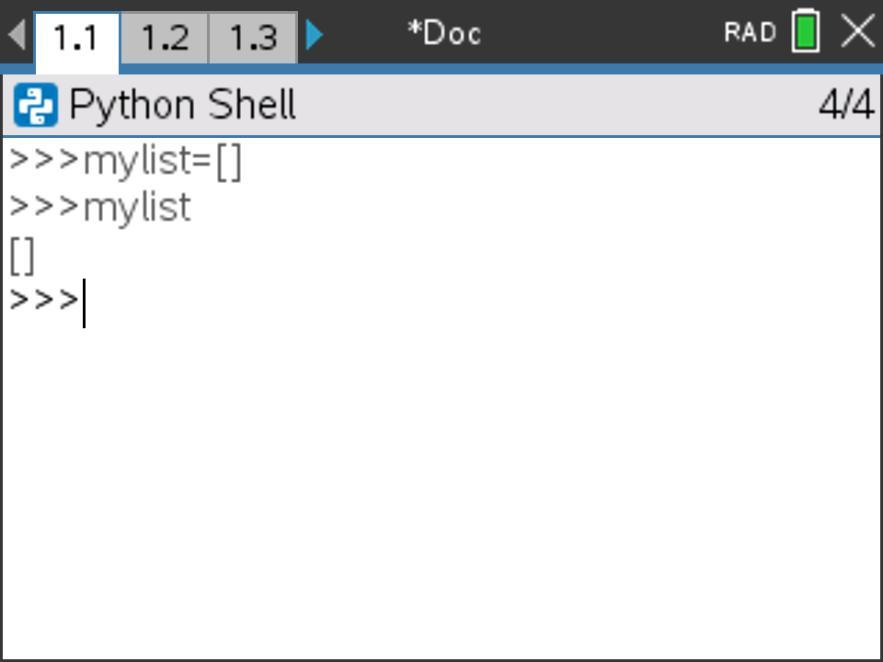We can also define mylist to be an array with data in it. Let’s redefine mylist to be an array containing the values 12, 7, and 2. (Think: How would you do that? Then check your thinking on the screenshot below.)

We can display the entire array mylist by again calling that array.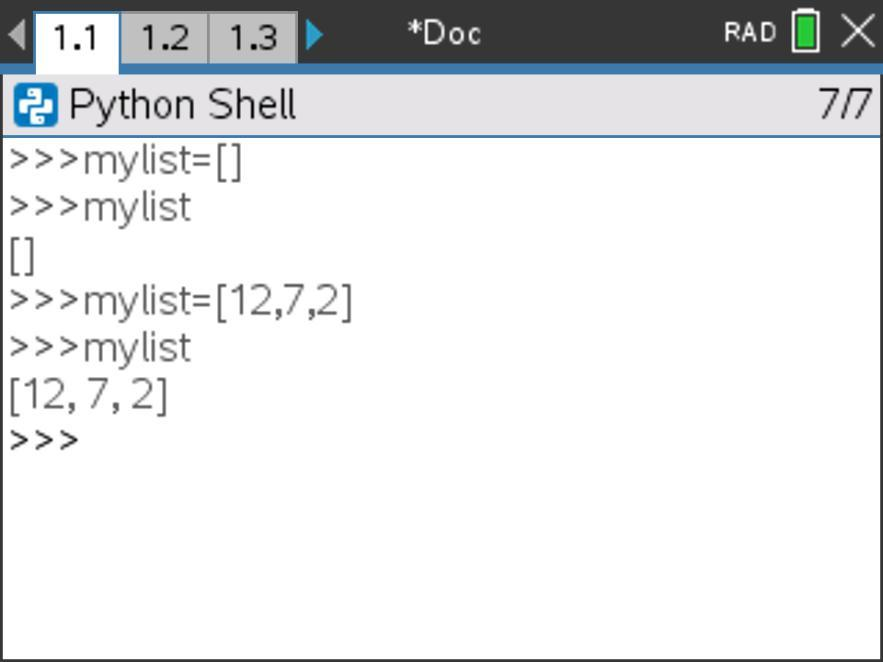However, sometimes we want to call or refer to a certain element in the array, not the entire array. We can do that with indexing, like this: mylist. What do you think will be displayed if we call mylist?

Let’s try it out and see.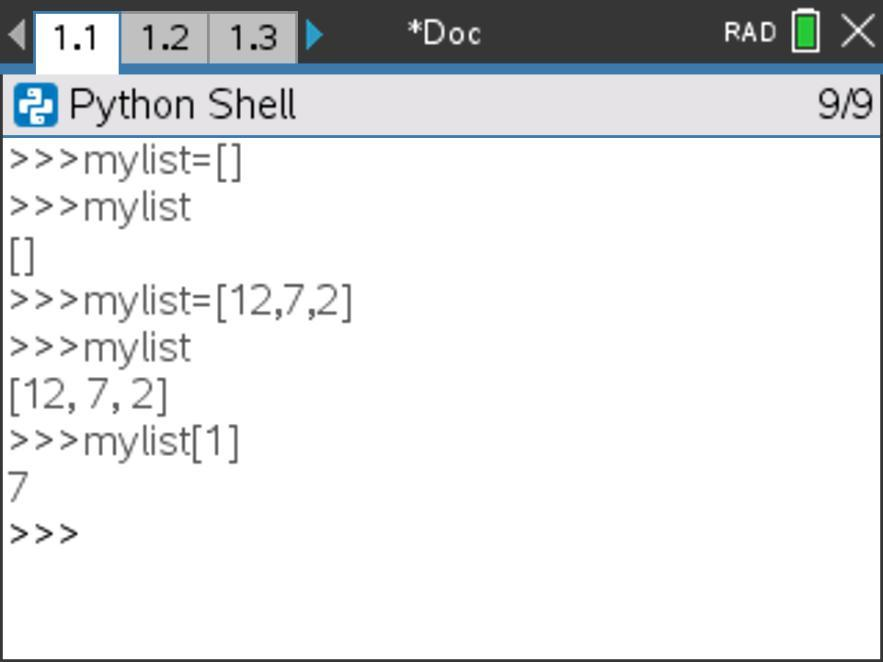This is an important nuance for us to consider. Notice that arrays in Python on the TI-Nspire™ platform are indexed starting with 0, meaning that the first element in an array is represented by index (or position) 0, not 1. This convention is used in many other coding languages, too. This phase shift is an important thing to keep in mind as we code out programs in the TI-Nspire™ CX II graphing calculator.

Let’s look at our whole array again. Let’s say we wanted to add to this array. If we call mylist to see what (if anything) is in element 3 in this array, we receive this error message: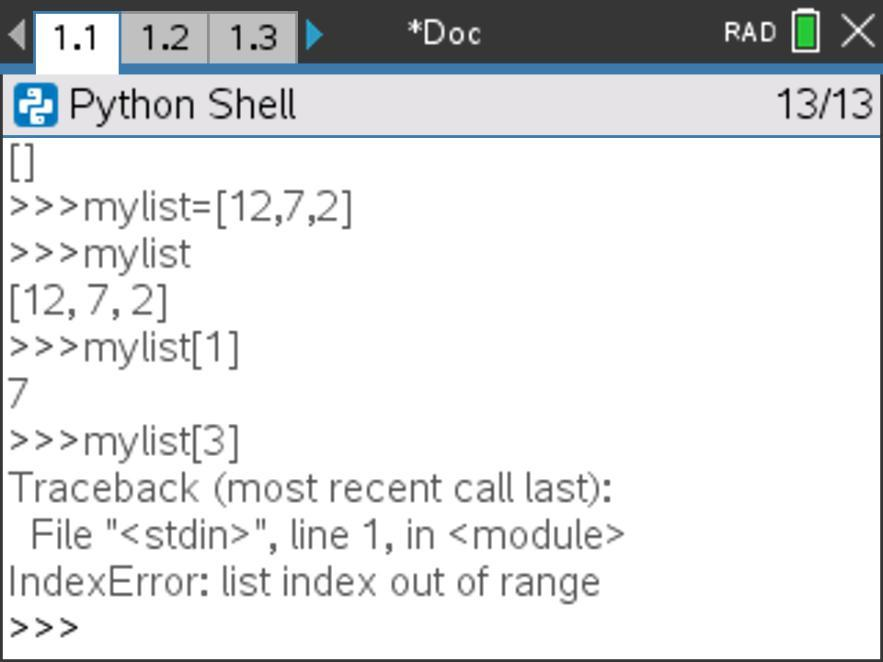This error message is tuned in to the exact problem with our mylist call—specifically, we can’t call the element in element 3 of mylist, because this list index (in other words, 3) is out of range. This list only contains elements in positions 0, 1 and 2.

Let’s try this line of code and see what happens: mylist.append(17). ). Now what do you predict will happen if we call mylist? What if we call mylist?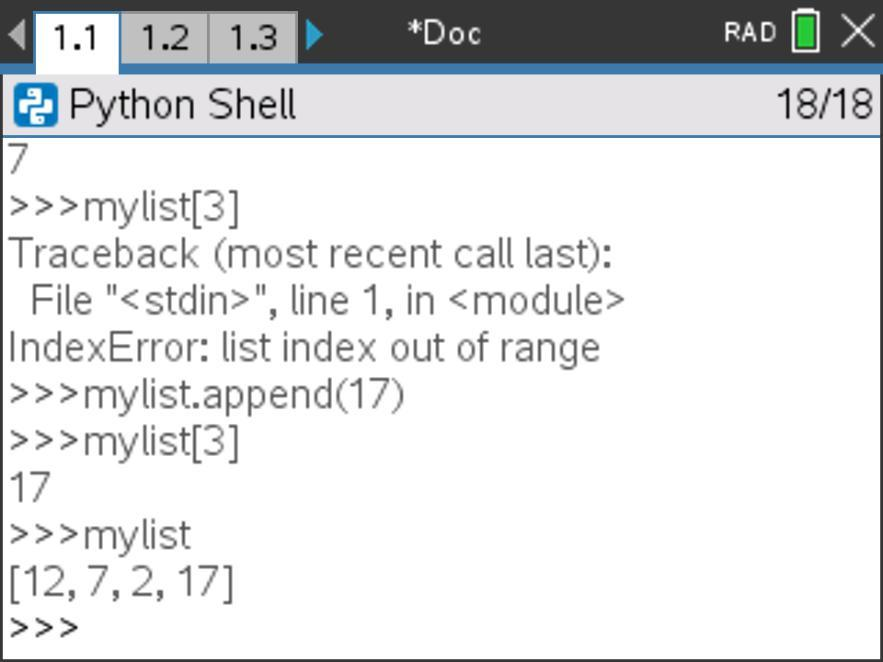Interesting! Now we see that the value 17 has been added to mylist in the third element. So, the command mylist.append(17) has added an element to the end of mylist and that element has a value of 17.

Tech tip: List commands can be found in Menu > Built-Ins > Lists.

Let’s look at one more command before we move into a programming space. What might this command return: len(mylist) ?

Let’s test it out and see.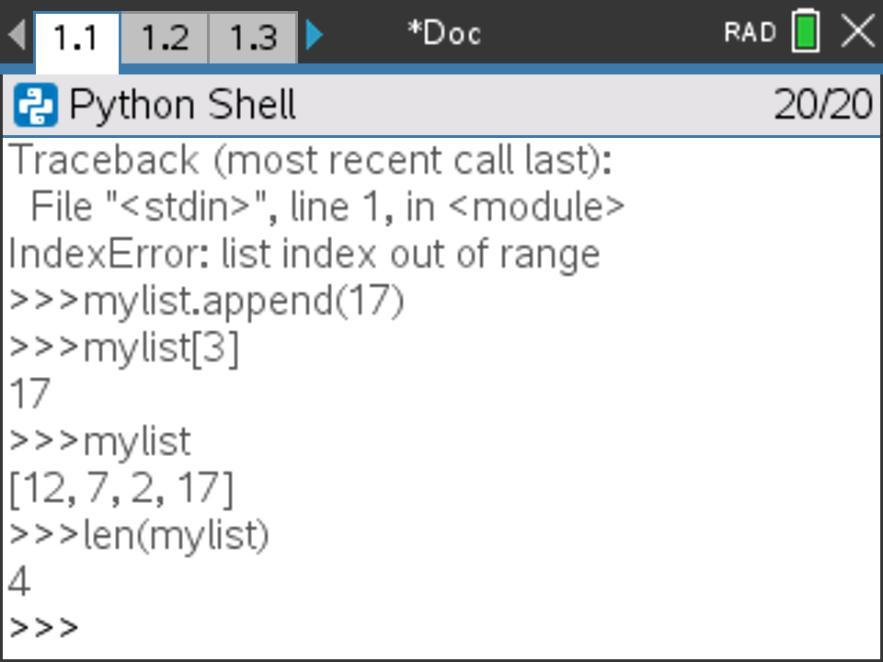It returns a 4. What does the len() command do? It returns the dimension or length of the array — the number of elements in the array. This will be a helpful command for us to use in many programs.

We’ve been exploring some array commands on Python Shell, but let’s now transition into a program interface and consider how these commands might be incorporated into various programs.

## Programming with lists in a Python program

To create a Python program, we can insert a Python page with a New program and then name our new program.

Take a look at the following program. Predict what the output will look like (line by line) when this program is executed.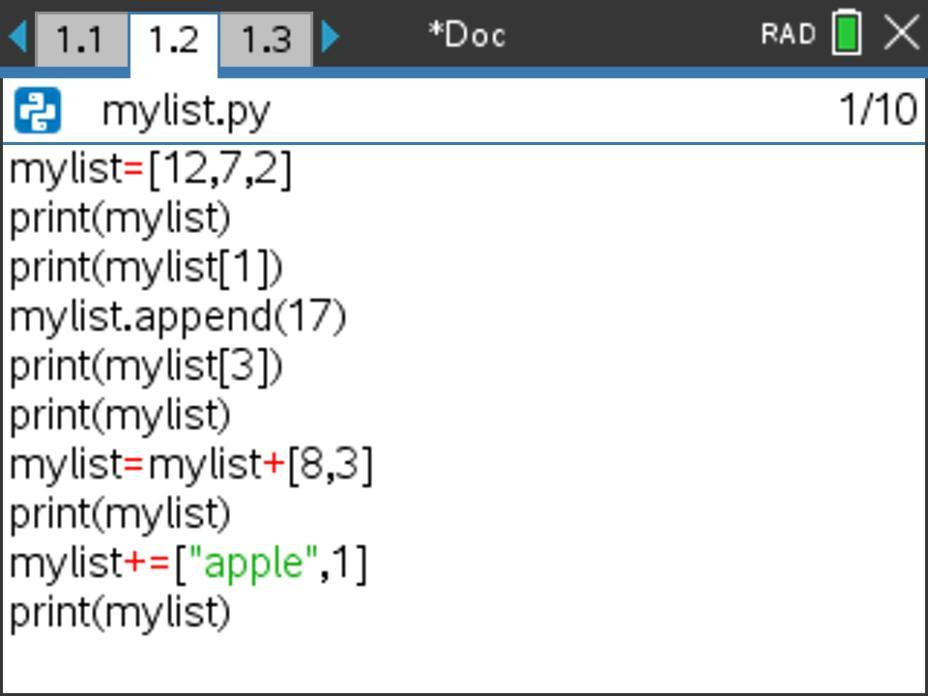Take a minute to trace through this program on your own. What do you predict the output of this program will be when we execute (or run) it?

Let’s execute this program to test our predictions.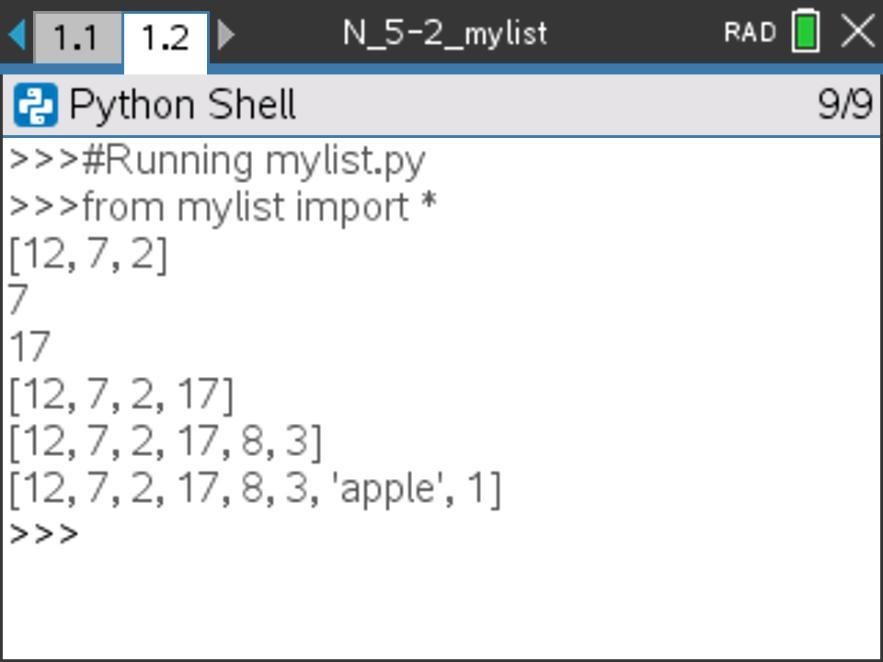How does the output of the program map to your prediction?

Notice that this program included two additional ways to add elements to the end of an array (other than .append() that we used earlier):

• mylist=mylist+[8,3] — Here we combine multiple arrays using the + operator
• mylist+=[‘apple’,1] — Here we add on to the element of a list using the += shorthand, incrementing operator. This is a condensed way to write mylist=mylist+[something].

Note: This same incrementing shorthand (+=) works for incrementing counters, too. c+=1 increments the variable c by 1.

## Formalizing 1D Arrays

Let’s use an interactive notes page to formalize the basics of creating, calling and adding to lists. Here are two pictures of the 1D Arrays Notes Page designed to capture our important learnings about arrays. This notes page uses the program mylist.py that you just traced through above.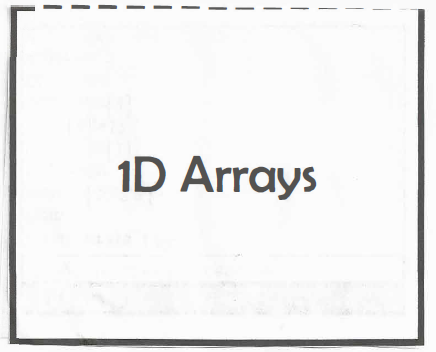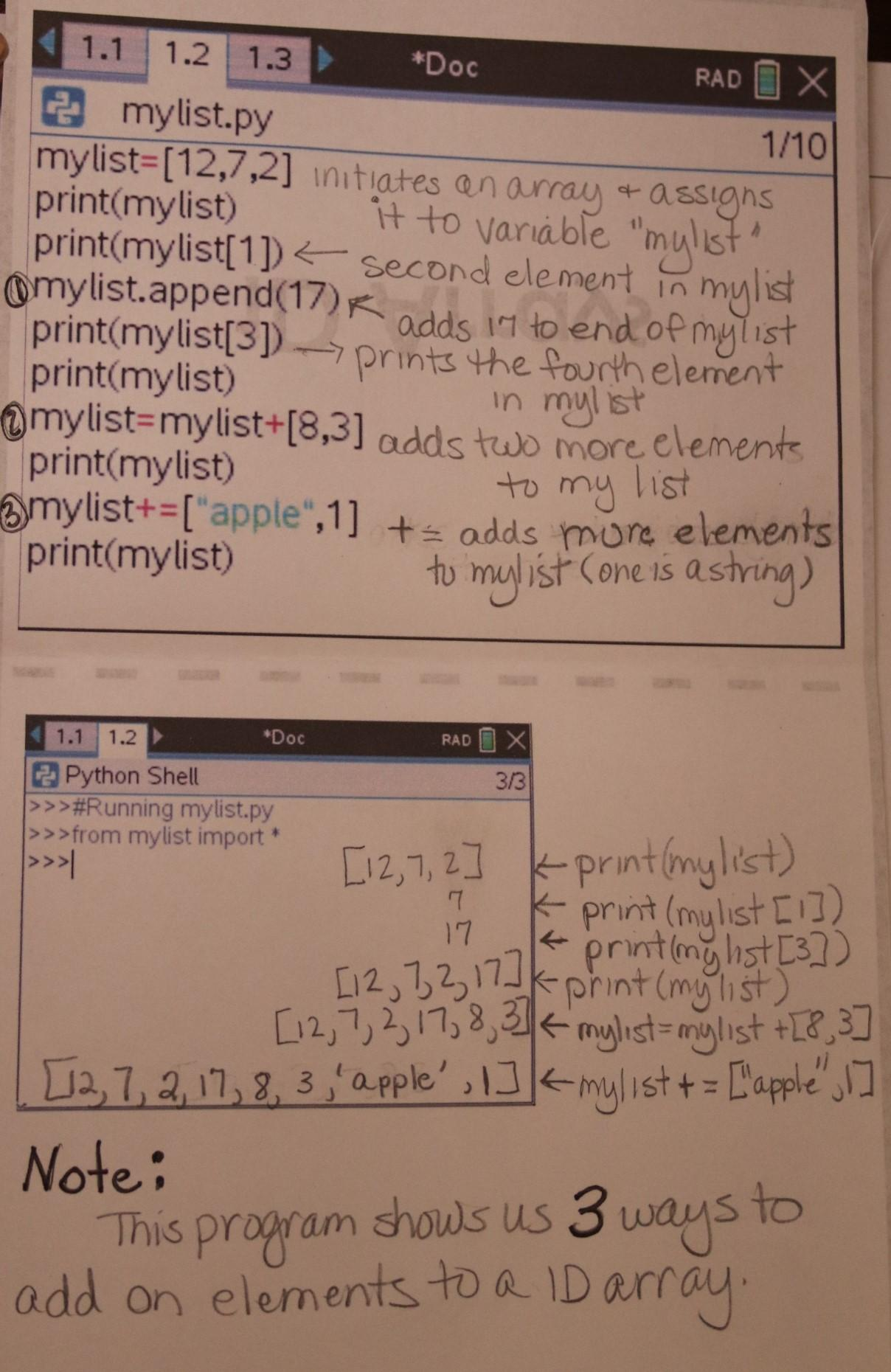## Displaying more elements in lists

Let’s explore one more idea before we move on from this mylist activity.

We know how to display an element in an array. Could we then write a program that would display every element in an array? What might that program look like?

Say we start with the array mylist=[12,7,2,17], then want to add in some lines of code to display each element in the array. We could do that like this:

• print(mylist)
• print(mylist)
• print(mylist)
• print(mylist)

That’s a lot of repetition, though. How else could we accomplish this task?

Since we’re doing a similar process again and again, we could abstract this process with a loop to make the code more efficient. In Python on the TI-Nspire™ CX II graphing calculator, the syntax for a For loop looks like this: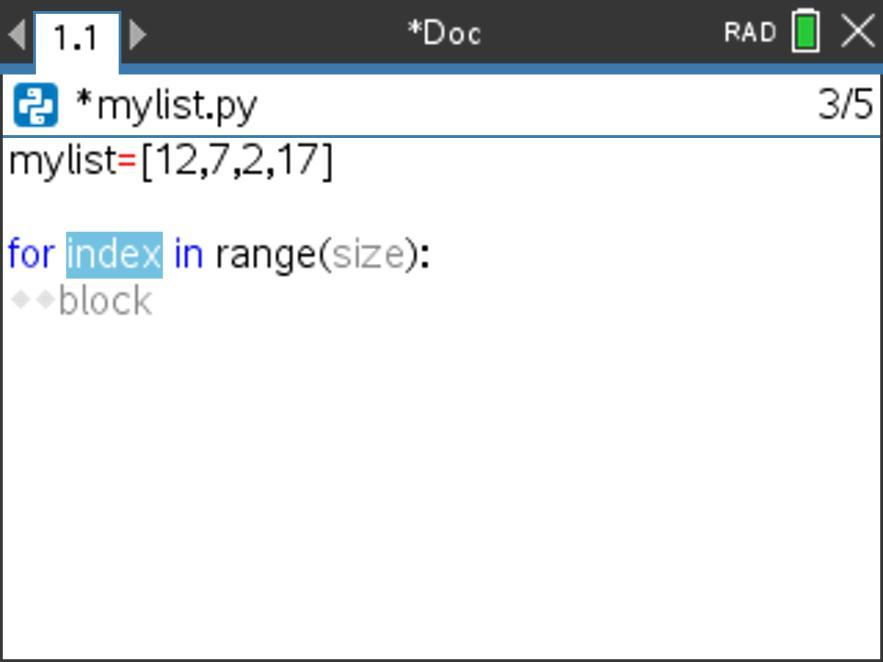Where index is where we add our loop variable (a variable that tracks our runs through the loop; often represented by i) and range(size) will increment from 0, 1, 2, 3, … up to the number before size. The default step value is 1.

If we want this loop to run through four times, we would update index and size as shown below. This will cause the loop to run four times: first i will have a value of 0, then i will have a value of 1, then 2, then 3. Note: the loop exits before i increments to 4.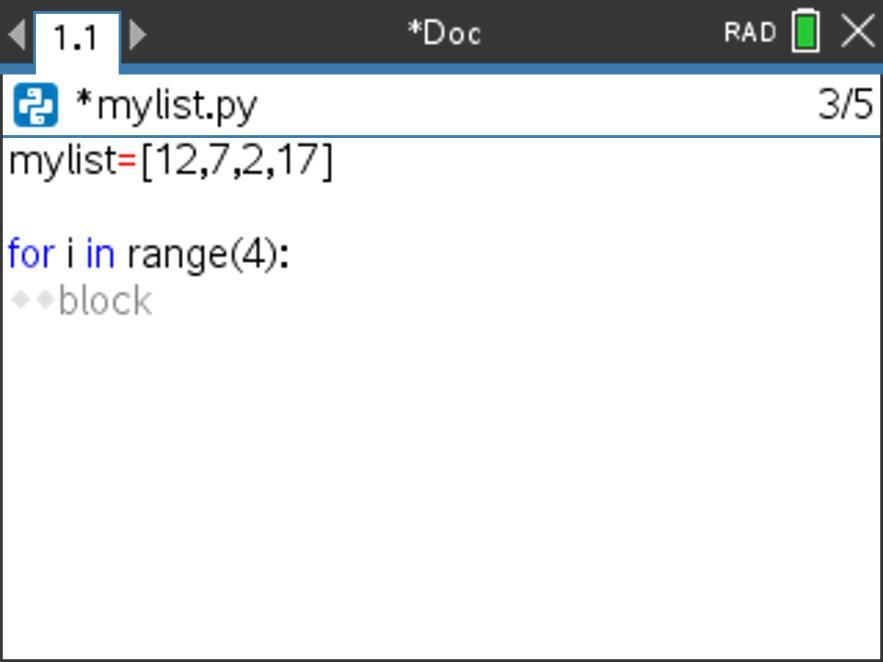What should be in the body of the loop?

We want the index we are calling within mylist to increment with each run through of the loop, so we’ll use print(mylist[i]) in order to loop through and print the various indexed elements of mylist.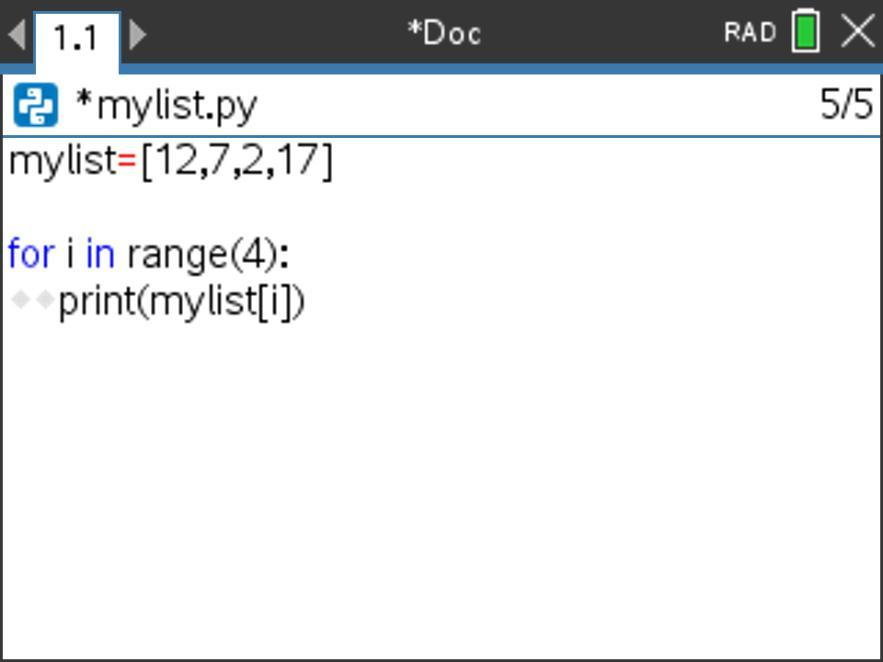Test out this code to see if it prints each individual element in mylist as desired!

Extension:

• How might you update the code so that it prints the elements in reverse order?

## Reflecting on programming with lists

In this activity, we explored the basics of using lists (also known as arrays) in programs, including creating and editing lists.

Consider:

• What might lists be useful for in programming? Why?

In the last activity in this series, will explore how to operate on lists and their elements and how to use these operations to analyze lists and their elements.

This series includes: